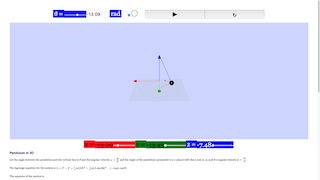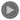Intro Page

This is a pendulum in 3D.

Let the angle between the pendulum and the vertical line is $$\theta$$ and the angular velocity $$\omega=\frac{d\theta}{dt}$$

And the angle of the pendulum (projected to x-y plane) with the x-axis is  $$\phi$$, and it's angular velocity $$\dot\phi=\frac{d\phi}{dt}$$

The [url=http://en.wikipedia.org/wiki/Lagrangian_mechanics]lagrange equation[/url] for the system is $$L=T-V = \tfrac{1}{2}m (L\dot\theta)^2+\tfrac{1}{2}m (L\sin\theta \dot{\phi})^2- (-mgL\cos\theta)$$

The equation of the motion is

$$\ddot\theta=\sin\theta\cos\theta\dot{\phi}^2-\frac{g}{L}\sin\theta$$  ...... from $$\frac{d}{dt}(\frac{\partial L}{\partial \dot{\theta}})-\frac{\partial L}{\partial \theta}=0$$

and

$$m L^2 \sin\theta^2 \dot{\phi}=const$$ Angular momentum is conserved. ...... from $$\frac{d}{dt}(\frac{\partial L}{\partial \dot{\phi}})-\frac{\partial L}{\partial \phi}=0$$

And the following is the simulation of such a system:

When the checkbox ([b]circular loop[/b]) is checked, $$\omega=0$$. and $$\dot{\phi}= \sqrt{\frac{g}{L\cos\theta}}$$ It is a circular motion.

The vertical component tangential of the string balanced with the mass m, and the horizontal component tangential provide the centripetal force for circular motion.

You can uncheck it and change the period $$T=\frac{2\pi}{\dot{\phi}}$$ ,

and you will find out the z-coordinate of the pendulum will change with time when

$$\omega\neq 0$$ or $$\dot{\phi}\neq \sqrt{\frac{g}{L\cos\theta}}$$

You can also drag the blue dot to change the length of the pendulum.

Pendulum 3D

### Translations

Code Language Translator Run### Software Requirements

SoftwareRequirements

 Android iOS Windows MacOS with best with Chrome Chrome Chrome Chrome support full-screen? Yes. Chrome/Opera No. Firefox/ Samsung Internet Not yet Yes Yes cannot work on some mobile browser that don't understand JavaScript such as..... cannot work on Internet Explorer 9 and below

### CreditsFu-Kwun Hwang - Dept. of Physics, National Taiwan Normal Univ.; lookang; tina

### end faq

http://iwant2study.org/lookangejss/02_newtonianmechanics_3dynamics/ejss_model_pendulum3D/pendulum3D_Simulation.xhtml

# Testimonials (0)

There are no testimonials available for viewing. Login to deploy the article and be the first to submit your review!